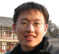### Lyons Zhang (@fpgaplayer)

Digital signal process, digital communication, FPGA engineer. He has been worked at Tieto company since 2011. He is a fan of Rick Lyons.

## 5G NR QC-LDPC Encoding Algorithm

3GPP 5G has been focused on structured LDPC codes known as quasi-cyclic low-density parity-check (QC-LDPC) codes, which exhibit advantages over other types of LDPC codes with respect to the hardware implementations of encoding and decoding using simple shift registers and logic circuits.

5G NR QC-LDPC  Circulant Permutation Matrix

A circular permutation matrix ${\bf I}(P_{i,j})$ of size $Z_c \times Z_c$ is obtained by circularly shifting the identity matrix $\bf I$ of...

## Polar Coding Notes: A Simple Proof

For any B-DMC $W$, the channels $\{W_N^{(i)}\}$ polarize in the sense that, for any fixed $\delta \in (0, 1)$, as $N$ goes to infinity through powers of two, the fraction of indices $i \in \{1, \dots, N\}$ for which $I(W_N^{(i)}) \in (1 − \delta, 1]$ goes to $I(W)$ and the fraction for which $I(W_N^{(i)}) \in [0, \delta)$ goes to $1−I(W)^{}$.

Mrs. Gerber’s Lemma

Mrs. Gerber’s Lemma provides a lower bound on the entropy of the modulo-$2$ sum of two binary random...

## Polar Coding Notes: Channel Combining and Channel Splitting

Channel Combining

Channel combining is a step that combines copies of a given B-DMC $W$ in a recursive manner to produce a vector channel $W_N : {\cal X}^N \to {\cal Y}^N$, where $N$ can be any power of two, $N=2^n, n\le0^{}$.

The notation $u_1^N$ as shorthand for denoting a row vector $(u_1, \dots , u_N)$.

The vector channel $W_N$ is the virtual channel between the input sequence $u_1^N$ to a linear encoder and the output sequence $y^N_1$ of $N$...

## Half-band filter on Xilinx FPGA

1. DSP48 Slice in Xilinx FPGA

There are many DSP48 Slices in most Xilinx® FPGAs, one DSP48 slice in Spartan6® FPGA is shown in Figure 1, the structure may different depending on the device, but broadly similar.

Figure 1: A whole DSP48A1 Slice in Spartan6 (www.xilinx.com)

2. Symmetric Systolic Half-band FIR

Figure 2: Symmetric Systolic Half-band FIR Filter

3. Two-channel Symmetric Systolic Half-band FIR

Figure 3: 2-Channel...

## Re: Question about Hogenauer's Paper

Hello Pro. Rick Lyons，Thank you for your magnificent fascinating blog https://www.dsprelated.com/showarticle/143.phpYes, you are right. The system function...

## Re: Question about Hogenauer's Paper

Hello Pro. Rick Lyons,Thank you very much for your incredibly fast replay.It seems that R in Eq.(3) indicates there is a downsampling R, for the Hc(z)=1-z^(-RM).I...

## Re: Digital signal processing

Dear Prof. Rick Lyons,Hope you are a handsome gentleman and happy everyday!I confused by Hogenauer's paper, could you help me?Question about Hogenauer's Paper (dsprelated.com)Thank...

Before I have not read Hogenauer's paper, but when I read I am confused about the paper. Could somebody help me?The denominator of (A2)，Here |z| = 1, why the second...

## Re: Off Topic: Binary Numbers

At least 2000 years ago, the primordial "Bagua" appeared. Don't know what's this? Haha...In China, crystal ball was useless, the wizard can predict everything...

## The MathJax CDN is shutting down on April 30, 2017!

https://www.mathjax.org/I don't believe April Fool joke.  :)

## Re: Source coding in WiFi or LTE

speech code : e.g. : AMR for 2G, 3G , 4G; AMR-WB for 4G(VoLTE)

## Re: Interpolate complement value

Thank you very much @drmike and @Tim Wescott, I am sorry I didn't say clearly, I mainly care about the Mix-Mode in the doc.complement.pngIn short, what are the...

## Interpolate complement value

I read a doc http://www.analog.com/media/en/technical-documenta... , page 49 AD9119_9129_page49.pdf , found an interesting implementation. I greatly appreciate If...

Use this form to contact fpgaplayer

Before you can contact a member of the *Related Sites:

• You must be logged in (register here)
• You must confirm you email address